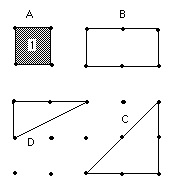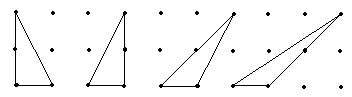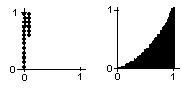## Sample Problems from Chapter 13

Chapter 13: Area Under Curves-- The Integral
1. Area on the geoboard.I start young people working on a geoboard. The geoboard I use is 1/2" plywood with a 5 by 5 square array of nails, about 2" apart with about 1" edge. I also have "spotty" paper, with dots the same distance apart as the geoboard so that my younger students can draw the shapes. I also have 1/2" spotty paper for the older students.
If it takes 1 can of paint to paint inside the square (figure A), how many cans of paint does it take to fill in figures B, C, and D?
There are many investigations one can get into: Make some shapes and find how many cans of paint it takes to paint inside them (in other words, find the area within each figure).
How many segments can you make on this 5x5 geoboard?
How many squares can you make on this 5x5 geoboard (no diagonal sides)?
What is the area of each triangle below?2. How Archimedes found the area within a parabolic segment - Not shown here.

3. Plotting points on a computer or programmable calculator to find the area under curves:I was browsing through a Scientific American "Computer Recreations" article in which they were filling in squares on a computer screen. I asked myself "Could I do that on our programmable graphing calculator?" After a couple of hours of trying things and making mistakes (I was never good at programming), I was able to plot points on the screen to make a square. Hoorah! I plotted points as in the figure at left below to make a 1x1 square. If you count the number of points, this number will be a measure of the area of the square!The next thing I asked of course was, could I find the area under a curve, say x2, as in the figure above at the right.
Write a program to plot points to make a square, then so it is possible to change the upper level of the points. Can you predict the area under y = x2?
Look at my program to plot the points.

4. Finding the area under curves on graph paperAt this time I realized that not every youngster had a programmable, graphing calculator. So I decided to use 1/10" graph paper to do the same thing.

First predict what part of the area of the 1x1 square at the right is under the curve y = x2. Graph the curve y = x2 carefully from x =0 to 2 (use 10 squares for 1 unit). Count the squares under the curve from 0 to 1, by approximating (carefully). Then count the the squares in the 1x1 square. Find the ratio of the no. of squares under the curve from 0 to 1, to the no. of squares in th 1x1 square. What simple fraction is close to this number?

Now count the squares under the curve from 0 to 2. Notice that the area surrounding the this part of the curve is not a square but a rectangle of 2*22 = 8 = 23. What fraction of this rectangle is under the curve?

5. The rectangle method (the standard textbook method) of finding the area under curves. - Not shown here.

6. Two problems. Two seemingly unrelated problems ended up with similar interesting solutions!
Problem 1. The area under the parabola y = x2, from 0 to 1.
Problem 2. The ratio of (the vol. of a square pyramid)/(the vol. of a cube), with the same base.
- this is not done here.
7. The natural logarithm is the area under a curve and an infinite series.
Fill in the table below. Guess the log 1 (read as the log of 1, and to base 10), first, and put your guess in the table in the right-hand column, even though you probably don't know what a log is! Then use a calculator to get the log 1, to 3 decimal places- put that in the center column, under 'calculator answer'. Then guess what the log 2 is. Put that number in the right-hand column. Use the clculator to get the log 2 to 3 decimal places- put that under 'calculator answer'. Fill in the rest of the table, and look for patterns as you go.

logs, to base 10       Calculator answer       write guess, first

log 1   =
log 2   =
log 3   =
log 4   =
log 5   =
log 6   =
log 7   =
log 8   =
log 9   =
log 10   =
log 20   =
log 200   =
log 2000   =
log 1/2   =
log 1/4   =
8. The area under the normal distribution curve. - not done here.
My program to plot points and other solutions to these kinds of problems
To choose sample problems from other chapters